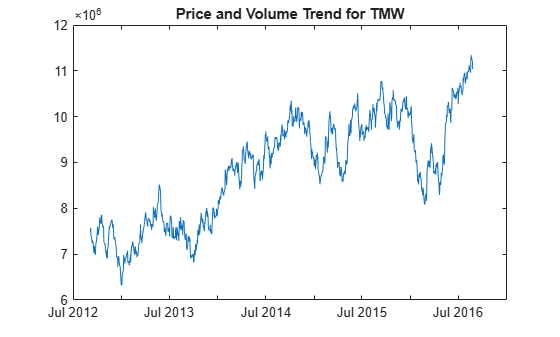Main Content

# pvtrend

Price and Volume Trend (PVT)

Using a `fints` object for the `Data` argument of `pvtrend` is not recommended. Use a matrix, `timetable`, or `table` instead for financial time series. For more information, see Convert Financial Time Series Objects fints to Timetables.

## Syntax

``trend = pvtrend(Data)``

## Description

example

````trend = pvtrend(Data)` calculates the Price and Volume Trend (PVT) from the series of closing stock prices and trade volume.```

## Examples

collapse all

Load the file `SimulatedStock.mat`, which provides a timetable (`TMW`) for financial data for TMW stock.

```load SimulatedStock.mat trend = pvtrend(TMW); plot(trend.Time,trend.PriceVolumeTrend) title('Price and Volume Trend for TMW')```## Input Arguments

collapse all

Data for closing prices and trade volume, specified as a matrix, table, or timetable. For matrix input, `Data` is an `M`-by-`2` matrix of closing prices and trade volume. Timetables and tables with `M` rows must contain variables named `'Close'` and `'Volume'` (case insensitive).

Data Types: `double` | `table` | `timetable`

## Output Arguments

collapse all

Price and volume trend (PVT), returned with the same number of rows (`M`) and the same type (matrix, table, or timetable) as the input `Data`.

 Achelis, S. B. Technical Analysis from A to Z. Second Edition. McGraw-Hill, 1995, pp. 239–240.

Download ebook